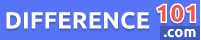Statistic

If we look at the statistic meaning, it can be defined as a numerical value obtained or drawn from the sample of the data. A statistic is a descriptive statistical measure and function of the sample observation. A sample can be defined as a fraction of the population that represents all the population’s characteristics. This sample is taken from the population to study, and the data produced by the perspective measured of that sample is the statistic. The sample has almost all the inferred population characteristics because it is impossible to study the entire population. Statistic is also famous for being a “sample statistic” because it is drawn from the sample. The sample statistic is used to collect information about every individual. However, the data may vary from sample to sample.